Courses

Test: 35 Year JEE Previous Year Questions: Gravitation

24 Questions MCQ Test Physics 35 Years JEE Main & Advanced Past year Papers | Test: 35 Year JEE Previous Year Questions: Gravitation

Description
Attempt Test: 35 Year JEE Previous Year Questions: Gravitation | 24 questions in 50 minutes | Mock test for JEE preparation | Free important questions MCQ to study Physics 35 Years JEE Main & Advanced Past year Papers for JEE Exam | Download free PDF with solutions
QUESTION: 1

STATEMENT - 1 : An astronaut in an orbiting space station above the earth experiences weightlessness. because STATEMENT - 2 :  An object moving the earth under the influence of Earth’s gravitational force is in a state of “freefall”.

Solution:

The normal force exerted by the astronaut on orbiting space station is zero (until the astronaut exerts some muscular force). Therefore the apparent weight of astronaut in an orbiting space station is zero. Astronaut is called in a state of weightlessness. This is because astronaut as well as space -ship are freely falling bodies.
Statement - 1 is true, statement - 2 is true and statement - 2 is the correct explanation of statement - 1.

QUESTION: 2

The kinetic energy needed to project a body of mass m from the earth surface (radius R) to infinity is

Solution: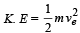where ve = escape velocity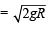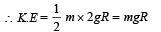QUESTION: 3

If suddenly the gravitational force of attraction between Earth and a satellite revolving around it becomes zero, then the satellite will

Solution:

Due to inertia of motion it will move tangentially to the original orbit in the same velocity.

QUESTION: 4

Energy required to move a body of mass m from an orbit of radius 2R to 3R is

Solution:

Energy required = (Potential energy of the Earth -mass system when mass is at distance 3R) – (Potential energy of the Earth -mass system when mass is at distance 2R)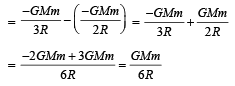QUESTION: 5

The escape velocity of a body depends upon mass as

Solution:

Escape velocity,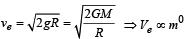Where M, R are the mass and radius of the planet respectively. In this expression the mass of the body (m) is not present showing that the escape velocity is independent of the mass.

QUESTION: 6

The time period of a satellite of earth is 5 hours. If the separation between the earth and the satellite is increased to 4 times the previous value, the new time period will become

Solution:

According to Kepler ’s law of planetary motion T2 ∝ R3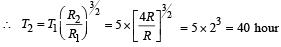QUESTION: 7

Two spherical bodies of mass M and 5M & radii R & 2R respectively are released in free space with initial separation between their centres equal to 12 R. If they attract each other due to gravitational force only, then  the distance covered by the smaller body  just before collision is

Solution:

The gravitational force acting on both the masses is the same. We know that

Force = mass × acceleration.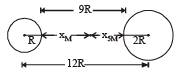For same force,   acceleration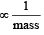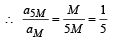.....(i)

Let t be the time taken for the two masses to collide and x5M, xM be the distance travelled by the mass 5M and M respectively.

For mass 5M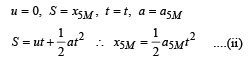For mass M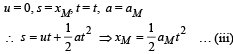Dividing (ii) by (iii)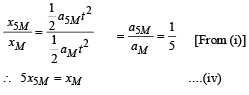From the figure it is clear that

x5M+ xM = 9R                ....(v)
Where O is the point where the two spheres collide.
From (iv) and (v)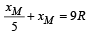∴ 6 xM= 45R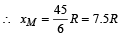QUESTION: 8

The escape velocity for a body projected vertically upwards from the surface of earth is 11 km/s. If the body is projected at an angle of 45°with the vertical, the escape velocity will be

Solution: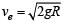The escape velocity is independent of the angle at which the body is projected.

QUESTION: 9

A satellite of mass m revolves around the earth of radius R at a height x from its surface. If g is the acceleration due to gravity on the surface of the earth, the orbital speed of the satellite is

Solution:

Gravitational force provides the necessary centripetal force.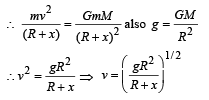QUESTION: 10

The time period of an earth satellite in circular orbit is independent of

Solution: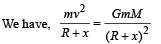x = height  of satellite from earth surface
m = mass of satellite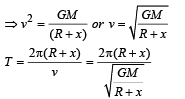which is independent of mass of satellite

QUESTION: 11

If ‘g’ is the acceleration due to gravity on the earth’s surface, the gain in the potential energy of an object of mass ‘m’ raised from the surface of the earth to a height equal to the radius ‘R' of the earth is

Solution: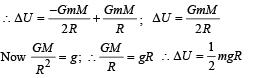QUESTION: 12

Suppose the gravitational force varies inversely as the nth power of distance. Then the time period of a planet in circular orbit of radius ‘R’ around the sun will be proportional to

Solution: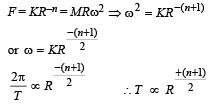QUESTION: 13

The change in the value of ‘g’ at a height ‘h’ above the surface of the earth is the same as at a depth ‘d’ below the surface of earth. When both ‘d’ and ‘h’ are much smaller than the radius of earth, then which one of the following is correct ?

Solution:

Variation of g with altitude is,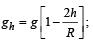variation of g with depth is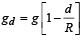Equating gh and gd , we get d = 2h

QUESTION: 14

A particle of mass 10 g is kept on the surface of a uniform sphere of mass 100 kg and radius 10 cm. Find the work to be done against the gravitational force between them to take the particle far away from the sphere  (you may take G = 6.67× 10-11 Nm2 / kg2)

Solution: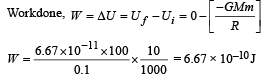QUESTION: 15

Average density of the earth

Solution: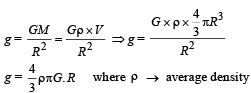QUESTION: 16

A planet in a distant solar system is 10 times more massive than the earth and its radius is 10 times smaller. Given that the escape velocity from the earth is 11 km s–1, the escape velocity from the surface of the planet would be

Solution: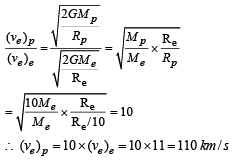QUESTION: 17

This question contains Statement-1 and Statement-2. Of the four choices given after the statements, choose the one that best describes the two statements.

Statement-1 : For a mass M kept at the centre of a cube of side ‘a’, the flux of gravitational field passing through its sides 4 π GM.

Statement-2: If the direction of a field due to a point source is radial and its dependence on the distance ‘r’ from the source is given
as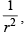its flux through a closed surface depends only on the strength of the source enclosed by the surface and not on the size or shape of the surface.

Solution:

Gravitational  flux through a closed surface is given by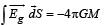where, M = mass enclosed in the closed surface

This relationship is valid when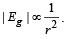QUESTION: 18

The height at which the acceleration due to gravity becomes 8/9 (where g = the acceleration due to gravity on the surface of the earth) in terms of R, the radius of the earth, is :

Solution: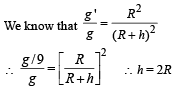QUESTION: 19

Two bodies of masses m and 4 m are placed at a distance r. The gravitational potential at a point on the line joining them where the gravitational field is zero is:

Solution:

Let the gravitational field at P, distant x from mass m, be zero.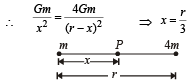Gravitational potential at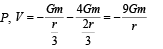QUESTION: 20

The mass of a spaceship is 1000 kg. It is to be launched from the earth's surface out into free space. The value of g and R (radius of earth) are 10 m/s2 and 6400 km respectively. The required energy for this work will be :

Solution:

The required energy for this work is given by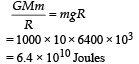QUESTION: 21

What is the minimum energy required to launch a satellite of mass m from the surface of a planet of mass M and radius R in a circular orbit at an altitude of  2R?

Solution:

Applying energy conservation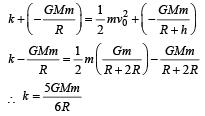QUESTION: 22

Four particles, each of mass M and equidistant from each other, move along a circle of radius R under the action of their mutual gravitational attraction. The speed of each particle is:

Solution: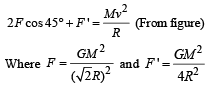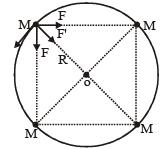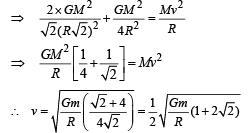QUESTION: 23

From a solid sphere of mass M and radius R, a spherical portion of radius R/2 is removed, as shown in the figure.
Taking gravitational potential V = 0 at r = ∞, the potential at the centre of the cavity thus formed is :

(G = gravitational constant)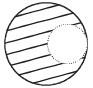Solution:

Due to complete solid sphere, potential at point P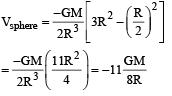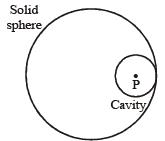Due to cavity part potential at point P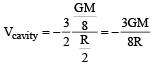So potential at the centre of cavity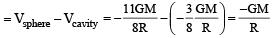QUESTION: 24

A satellite is revolving in a circular orbit at a height 'h' from the earth's surface (radius of earth R; h < < R). The minimum increase in its orbital velocity required, so that the satellite could escape from the earth's gravitational field, is close to : (Neglect the effect of atmosphere.)

Solution:

For h << R, the orbital velocity is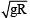Escape velocity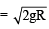∴ The minimum increase in its orbital velocity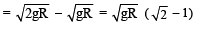Use Code STAYHOME200 and get INR 200 additional OFF Use Coupon Code

Track your progress, build streaks, highlight & save important lessons and more!

Similar ContentRelated tests